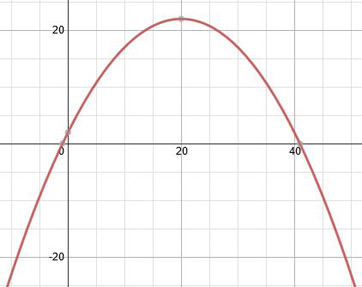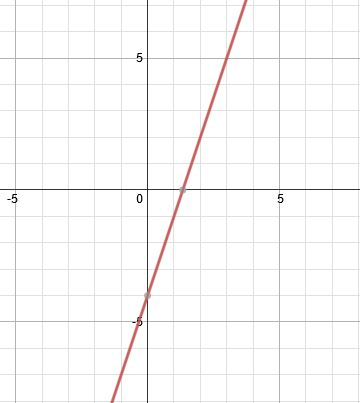# Short Run & Long Run Behavior of Polynomials: Definition & Examples

An error occurred trying to load this video.

Try refreshing the page, or contact customer support.

Coming up next: How to Graph Cubics, Quartics, Quintics and Beyond

### You're on a roll. Keep up the good work!

Replay
Your next lesson will play in 10 seconds
• 0:01 Behavior of Polynomials
• 1:25 Short Run Behavior
• 2:14 Long Run Behavior
• 2:55 Examples
• 5:02 Lesson Summary

Want to watch this again later?

Timeline
Autoplay
Autoplay
Speed

#### Recommended Lessons and Courses for You

Lesson Transcript
Instructor: Yuanxin (Amy) Yang Alcocer

Amy has a master's degree in secondary education and has taught math at a public charter high school.

Watch this video lesson to learn how you can use the graph of a polynomial to help you explain the polynomial function. Learn how to explain the behavior of the polynomial at the origin and at the far ends.

## Behavior of Polynomials

Polynomial functions are functions made up of terms composed of constants, variables, and exponents, and they're very helpful. They help us describe events and situations that happen around us. For example, the polynomial function f(x) = -0.05x^2 + 2x + 2 describes how much of a certain drug remains in the blood after x number of hours. Graphing this medical function out, we get this graph:Looking at the graph, we see the level of the drug first increases and then decreases over time. After about 41 hours or so, the drug is fully gone from the blood. In math, we have two ways of describing our graph. The first is called short run behavior, which is the behavior of the graph around the origin, and the second is called long run behavior, which is the behavior of the graph at the far ends of the graph.

Let's look at these now with our medical graph. Mathematicians like to look into the behavior of polynomials at these two areas because it gives them an idea of what happens to functions at their extremes. This helps with making hypothetical predictions about what might happen when an extreme circumstance occurs.

## Short Run Behavior

Let's look first at the short run behavior. Again, this is the behavior around the origin. Looking at our graph, we see that around the origin, when we have an x value of 0, we already have some of the drug in the blood. In terms of what is really happening, at 0 hours, the patient received the shot of the drug.

Theoretically, according to the function, if we go back in time just a bit, the drug level in the blood will decrease to 0. If we go a bit further, the drug level drops to negative. We know in reality that after the drug's level reaches 0, it can't physically drop any farther. We also see that if we go a bit to the right, the drug's level in the blood increases. So, we see that the short run behavior of this function is that it increases over time.

## Long Run Behavior

The next behavior is the long run behavior. This is the behavior at the far ends of the graph. When I say far ends, I mean the far left and far right. Looking at our graph, we see that as we go to the far left and to the far right, the graph goes down to negative infinity. So, theoretically, the blood level of the drug just keeps dropping. So, we can say that the long run behavior of the drug is that it goes down to negative infinity. This is the kind of analysis that is required when looking at short run and long run behavior. Let's look at a couple more graphs.

## Examples

This is a graph of the function f(x) = 3x - 4:Looking close to the origin, which is the point (0, 0), we see that our graph intersects the y-axis at -4. We also see that the graph steadily increases around the origin as well. There we have the short run behavior. As for the long run behavior, if we go to the far left, we see that the graph goes down to negative infinity. Going to the far right, we see that the graph goes up to positive infinity.

To unlock this lesson you must be a Study.com Member.

### Register to view this lesson

Are you a student or a teacher?

### Unlock Your Education

#### See for yourself why 30 million people use Study.com

##### Become a Study.com member and start learning now.
Back
What teachers are saying about Study.com

### Earning College Credit

Did you know… We have over 160 college courses that prepare you to earn credit by exam that is accepted by over 1,500 colleges and universities. You can test out of the first two years of college and save thousands off your degree. Anyone can earn credit-by-exam regardless of age or education level.